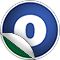# EquationConj

### Related Docs: class EquationConj | package equations

#### object EquationConj

Linear Supertypes
AnyRef, Any
Ordering
1. Alphabetic
2. By inheritance
Inherited
1. EquationConj
2. AnyRef
3. Any
1. Hide All
2. Show all
Visibility
1. Public
2. All

### Value Members

1. #### final def !=(arg0: Any): Boolean

Definition Classes
AnyRef → Any
2. #### final def ##(): Int

Definition Classes
AnyRef → Any
3. #### final def ==(arg0: Any): Boolean

Definition Classes
AnyRef → Any

9. #### def apply(lhss: Iterable[LinearCombination], order: TermOrder): EquationConj

Create an equation conjunction from an arbitrary set of equations (left-hand sides).

11. #### def apply(lhss: Iterator[LinearCombination], modEquations: ReduceWithEqs, logger: ComputationLogger, order: TermOrder): EquationConj

Create an equation conjunction from an arbitrary set of equations (left-hand sides), module another set of equations

13. #### def apply(lhss: Iterator[LinearCombination], logger: ComputationLogger, order: TermOrder): EquationConj

Create an equation conjunction from an arbitrary set of equations (left-hand sides).

14. #### final def asInstanceOf[T0]: T0

Definition Classes
Any
15. #### def clone(): AnyRef

Attributes
protected[java.lang]
Definition Classes
AnyRef
Annotations
@throws( ... )

19. #### def conj(conjs: Iterator[EquationConj], logger: ComputationLogger, order: TermOrder): EquationConj

Compute the conjunction of a number of systems of equations.

Compute the conjunction of a number of systems of equations. TODO: This could be optimised much more.

20. #### def createFromReducedSeq(lhss: Seq[LinearCombination], order: TermOrder): EquationConj

Create an equation conjunction from a canonised, reduced and sorted set of equations (left-hand sides).

21. #### final def eq(arg0: AnyRef): Boolean

Definition Classes
AnyRef
22. #### def equals(arg0: Any): Boolean

Definition Classes
AnyRef → Any
23. #### def finalize(): Unit

Attributes
protected[java.lang]
Definition Classes
AnyRef
Annotations
@throws( classOf[java.lang.Throwable] )
24. #### final def getClass(): Class[_]

Definition Classes
AnyRef → Any
25. #### def hashCode(): Int

Definition Classes
AnyRef → Any
26. #### final def isInstanceOf[T0]: Boolean

Definition Classes
Any
27. #### final def ne(arg0: AnyRef): Boolean

Definition Classes
AnyRef
28. #### final def notify(): Unit

Definition Classes
AnyRef
29. #### final def notifyAll(): Unit

Definition Classes
AnyRef
30. #### final def synchronized[T0](arg0: ⇒ T0): T0

Definition Classes
AnyRef
31. #### def toString(): String

Definition Classes
AnyRef → Any
32. #### final def wait(): Unit

Definition Classes
AnyRef
Annotations
@throws( ... )
33. #### final def wait(arg0: Long, arg1: Int): Unit

Definition Classes
AnyRef
Annotations
@throws( ... )
34. #### final def wait(arg0: Long): Unit

Definition Classes
AnyRef
Annotations
@throws( ... )